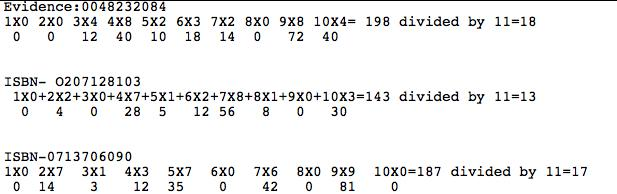#### You may also like### Prompt Cards

These two group activities use mathematical reasoning - one is numerical, one geometric.### Consecutive Numbers

An investigation involving adding and subtracting sets of consecutive numbers. Lots to find out, lots to explore.### Exploring Wild & Wonderful Number Patterns

EWWNP means Exploring Wild and Wonderful Number Patterns Created by Yourself! Investigate what happens if we create number patterns using some simple rules.

# Book Codes

##### Age 7 to 11 Challenge Level:
This is quite a challenging question. However we had some good reports of what you did sent in. Fristly from Kirin at Carrington Primary School who sent in the following:

To solve the problem you must add up all  the times table questions then divide your answer by eleven.Alissa from Brabyns Prep School sent in this;

Eight classmates and I tried the procedure for ten books each.  My friends couldn’t find anything that the results had in common but I noticed that all my results were three-digit numbers where if you add the hundreds digit and the units digit you get the tens digit e.g. 242.

We checked my theory with my friends’ results and found that it mostly worked.  However, a few times we saw the number 209, which didn’t fit with my theory.  Also, one of my friends had a total of 77 which is not a three-digit number, but would still fit the theory as you could consider the hundreds digit to be 0.

We talked as a group and remembered something about a divisibility rule for 11 and when we checked we realised that all our numbers were divisible by 11.  There were about five other answers which didn’t fit my theory, but when we checked these, the calculations had been done incorrectly!

We could then work out the missing digit from the ISBN code in the problem. We used trial and error to find the missing digit, 8.  This made the total sum 264.  It was the only possible solution.

We didn’t really know why ISBN numbers needed to be coded, but we thought maybe it was something to do with security, regulation and copyright issues?

We REALLY enjoyed working on this problem.

Owl Class from Culham Parochial Primary School sent in this description of their work;

Firstly we all worked out an ISBN number and collated all of out results. We then began to look for things that they had in common.

We decided to see what they were all divisible by and whether they were all divisible by a common number. We recognised that it was not worth trying to divide the numbers by even numbers (such as 2,4 or 6) as answers were both odd and even and all multiples of even numbers must be even.

We then checked the 3 times table by adding the digits in the answer together and seeing if the answer was a multiple of 3 - not all of them were.

We then checked the 3 times table by adding the digits in the answer together and seeing if the answer was a multiple of 9 - not all of them were.

We checked to see if they were in the 5 times table by seeing if the number ended in a 5 or a 0 - not all of them did.

We then started to check the times tables we had no pattern for with a calculator - we discovered that all of the answers were divisible by 11.  We carried on to see if we could find any others, but we could could not find any more.

Well done all of you. I hope that ALL of you found it really enjoyable! Keep sending in your solutions to other activities.